Thank you for visiting nature.com. You are using a browser version with limited support for CSS. To obtain the best experience, we recommend you use a more up to date browser (or turn off compatibility mode in Internet Explorer). In the meantime, to ensure continued support, we are displaying the site without styles and JavaScript.

# Impact of an elastic sphere with an elastic half space revisited: Numerical analysis based on the method of dimensionality reduction

## Abstract

An impact of an elastic sphere with an elastic half space under no-slip conditions (infinitely large coefficient of friction) is studied numerically using the method of dimensionality reduction. It is shown that the rebound velocity and angular velocity, written as proper dimensionless variables, are determined by a function of only the ratio of tangential and normal stiffness ("Mindlin-ratio"). The obtained numerical results can be approximated by a simple analytical expression.

## Introduction

Impacts of solid particles are of interest for many physical and technological processes related to the dynamics of granular media1,2,3,4. Even if the particles have a spherical shape and the material is purely elastic, the detailed dynamics of the impact can be very complicated and include partial slip, gross slip or no slip in the contact area during different phases of the impact. This is the reason why a comprehensive theory of frictional impacts has not been developed so far. The exact analytical solution exists only for the simplest case when complete sliding (gross slip) occurs in the whole contact area during the entire impact. However, already for the other limiting case of no slip in the whole contact area (infinitely large coefficient of friction) the complete solution has not been obtained so far in spite of the apparent simplicity of the problem. The classical theory using only the conservation laws and the rolling conditions, which can be found in textbooks on mechanics5, is intrinsically inconsistent as it considers the impact as being elastic but at the same time uses the condition of rigid rotation of the body as a whole. The tangential compliance of the contact as well as micro slip in the contact area has been taken into account first by Maw, Barber and Fawcett6 (MBF theory), based on the theory of normal contact for elastic spheres by Hertz7 and the theory by Mindlin for tangential contacts8. Barber later provided an analytical theory for only those phases of the impact during which complete adhesion takes place9. The MBF theory was validated experimentally by the authors themselves10 as well by other authors11,12,13,14. A review of existing impact models and their validation can be found in the book15. In spite of the long history of studies of impacts, the existing results still cover only part of the theoretically possible impact parameters and no effective numerical methods for analyzing impact processes could be developed so far.

In the present paper we consider one of the simplest cases of an impact: an impact with no-slip in the contact area during the whole time of contact. Theoretical foundation for the solution of this problem was created by Hertz6 and Mindlin8 and is used in the MBF theory. The reason why this problem still has not been studied exhaustively is the mathematical complexity of the combined, time dependent normal and tangential problem. In a series of recent papers, Popov et. al. have shown that the theory by Hertz-Mindlin can be reproduced exactly by a contact of properly modified profile shape with a linear elastic foundation consisting of independent springs16,17,18,19,20. The method can be used not only for the combined normal-tangential contact with arbitrary history of loading but also for the rolling contact21. This method, called method of dimensionality reduction, MDR, simplifies the contact problem drastically and opens new ways for analytical and numerical treatment of dynamic normal and tangential contacts.

The paper is organized as follows. We first reproduce, for comparison with known exact results, the classical solution based on the assumption of rigid rotation at the last moment of contact. We then solve a simplified model with a constant contact stiffness, which provides the general understanding of the problem and the dimensionless variables which are of interest and will be used in the following analysis. After this the impact problem is solved using the method of dimensionality reduction.

## Results

### Simplified model of the impact with no-slip condition

#### Classical “rigid body” solution

Let us consider an impact of an elastic sphere with mass m and radius R on an elastic half space, as shown in Fig. 1. Let the moduli of elasticity of the sphere and the half space be E1 and E2, their Poison's numbers ν1 and ν2 and their shear moduli G1 and G2, accordingly. The main notations are illustrated in Fig. 1: The incident velocity of the center of mass of the sphere is ν0 with horizontal and vertical components νx0 and νz0, the incident angular velocity ω0, the rebound velocity is ν with components νx and −νz0, the grazing angle is α and the rebound angle β.

We first reproduce the classical solution of the impact problem. Let Fx and Fz be the components of contact force acting on the sphere during the impact. The equations of motion of the sphere in the integral form can be written aswhere t is the duration of the impact and I = (2/5)mR2 is the moment of inertia of a homogeneous sphere. Together with the rolling condition for the tangential rebound velocity,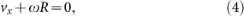these equations determine unambiguously all kinematic quantities of the sphere after the impact: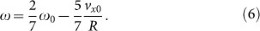It can be easily seen that the impact is non-elastic, as the energy change during the impact,is negative. This solution is, however, oversimplified. While equations (1)(3) are exact (under assumption of very short impact time), the kinematic condition (4) is intrinsically controversial: it cannot be valid during the whole time of impact and its application to the last moment of impact is an arbitrary and not substantiated assumption. In reality, due to the elasticity of the sphere, the condition (4) will be valid only at one point in time during the impact.

#### Impact in the case of a constant contact stiffness

In a second step let us take into account the normal and tangential compliance of the contact in a simplified way. The normal and tangential compliance of the contact are changing during the impact due to changing contact configuration. Let us simplify this situation by considering an impact of a rigid sphere having a linear spring in the contact region. This also can be an elastic sphere with a flat patch. Due to the flat the contact stiffness will be constant provided the contact radius does not change considerably during the impact. The considered system and notation are shown in Fig. 2.

Equations of motion can be written as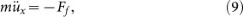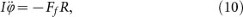where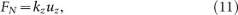are the normal and tangential components of the contact force. In the last equation we took into account the fact that the tangential displacement of the contact point is a sum of the displacement of the center of mass and the displacement due to rigid rotation. The solution of the set of Eq. (8)(12) with the initial conditions ux(0) = 0,, uz(0) = 0,, ϕ(0) = 0,has the form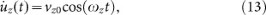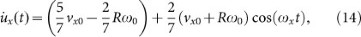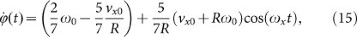whereand. The duration of the impact, ti, is determined by the Eq. (13) and is equal to ti = π/ωz. The velocities at the last moment of the impact are equal to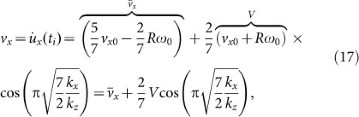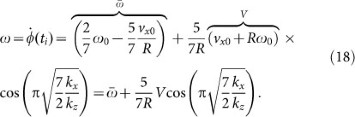The energy change during the impact is equal to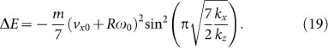Note that the expressions forandare exactly the classical solutions (5) and (6), while the remainder of Eq. (17) and (18) describes the influence of the finite tangential compliance. From Eq. (17) and (18), it follows that the combinations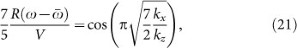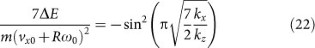are functions of the ratio kx/kz of the normal and tangential contact stiffness. This suggests that the structure of the relations (20) and (21) may be valid for a more general case of contact of any shape. Indeed, for an arbitrary rotationally symmetric body the ratio of differential tangential and normal stiffnesses is constant and equal to Ref. 8 kx/kz = G*/E*, where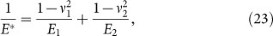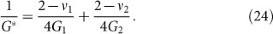In Refs. 22 and 23 it was shown by numerical simulations that this is valid even for randomly rough fractal surfaces. We thus may anticipate that the Eq. (20) and (21) are valid for both regular forms and rough profiles, while the exact dependence may be replaced by another, shape dependent function. We arrive at the hypothesis that in the general case the relations (20) and (21) have to by replaced by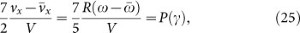whereIn the next Section, we will prove this hypothesis by numerical simulations and find the form of the function P(γ).

### Impact of a sphere: results of modeling

In the present work, the equations of motion (8)–(10) were solved by the Euler integration procedure. The results for the energy change during the impact as a function of the parameter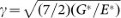are presented in Fig. 3, where the dimensionless variables,andare plotted as a function of the parameter γ defined by Eq. (27). Note that if the bodies have equal Poisson numbers: ν1 = ν2 = ν, then G*/E* = 2(1 − ν)/(2 − ν). From the thermodynamic stability criterion, it follows that Ref. 24 −1 < ν ≤ 1/2. Thus, for isotropic bodies, 2/3 < G*/E* < 4/3 which corresponds toHowever, for anisotropic (e.g. orthotropic) media, the effective ratio G*/E* can be in a wider range than given by this Equation. We therefore present results outside the region (28) as well.

Fig. 4 is a magnified representation of the most practically relevant range of the variable γ. In this range the numerically determined function P(γ) can be approximated withwith a = 0.195, b = 0.061 and k = 1.685. For practically important case of ν = 1/3 we get P ≈ 0.20 and for incompressible media (ν = 1/2), P ≈ −0.09.

## Discussion

In the present paper, we used the method of dimensionality reduction in the area of its exact applicability (contact of axis-symmetric bodies) to simulate an impact of an elastic sphere on an elastic half-space. The main result of the study is the proof of the hypothesis (25) as well as numerical determination of the function P(γ) appearing in this equation. This function is presented in Fig. 3. A simple analytical approximation (29) was found for this function. We investigated a much wider range of the ratios G*/E* than would be relevant for isotropic elastic bodies. As for anisotropic bodies (e.g. media with orthotropic elasticity), this ratio can in principle have arbitrary values. The suggested method can be generalized straightforwardly to impacts of bodies of different form (not necessarily spherical), impact with adhesion, contacts with dry friction or impact of viscoelastic bodies.

## Methods

For simulation of normal and tangential contact during the impact we use the method of dimensionality reduction (MDR). In the framework of the MDR, two preliminary steps are performed19: First, the three-dimensional elastic bodies are replaced by a one-dimensional linearly elastic foundation consisting of an array of independent springs, with a sufficiently small separation distance Δx and normal and tangential stiffness Δkz = Ex and Δkx = Gx. In the second step, the three-dimensional profile z = f(r) is transformed into a one-dimensional profile g(x) according toWhen the MDR-transformed profile g(x) is indented into the elastic foundation and is moved normally and tangentially according to an arbitrary law, the force-displacement relations of the equivalent one-dimensional system will reproduce those of the initial three-dimensional contact problem (proofs have been done in Refs. 18, 19.) The MDR solution has the same accuracy as the solutions by Cattaneo25 and Mindlin8: in the case of general Poisson ratio, there is an inaccuracy, which has been shown to be generally quite small26.

For a sphere with radius R, the shape in the vicinity of the contact is given by f(r) = r2/(2R). The one-dimensional MDR-image, according to (30), is g(x) = x2/R. If the vertical displacement uz of the center of mass is counted from the moment of first contact, it coincides with the indentation depth and the vertical displacement of the spring of the elastic foundation at the point xi,, is equal toinside the contact region. The contact radius a is determined by the condition. Due to the assumed stick condition in the whole contact region, the temporal incremental changesof the tangential displacements of all springs that are in contact with the profile, are equal to the rigid-body movement of the contact point,. The normal and tangential forces are calculated easily as,, where summation is over all springs in contact at the given moment of time.

## References

1. Thornton, C. & Yin, K. K. Impact of elastic spheres with and without adhesion. Powder Technology 65, 153–166 (1991).

2. Ciamarra, M. P. et al. Dynamics of drag and force distributions for projectile impact in a granular medium. Phys. Rev. Lett. 92, 194301 (2004).

3. Jop, P., Forterre, Y. & Pouliquen, O. A constitutive law for dense granular flows. Nature 441, 727–730 (2006).

4. Brilliantov, N. V., Spahn, F., Hertzsch, J.-M. & Pöschel, T. Model for collisions in granular gases. Phys. Rev. E 53, 5382–5392 (1996).

5. Hauger, W., Schnell, W. & Gross, D. Technische Mechanik. Bd 3: Kinetik 7 [149–150] (Springer, Auflage2002).

6. Maw, N., Barber, J. R. & Fawcett, J. N. The oblique impact of elastic spheres. Wear 38, 101–114 (1976).

7. Hertz, H. Über die Berührung fester elastischer Körper. Journal für die reine und angewandte Mathematik 92, 156–171 (1882).

8. Mindlin, R. D. Compliance of elastic bodies in contact. J. of Applied Mechanics 16, 259–268 (1949).

9. Barber, J. R. Adhesive contact during the oblique impact of elastic spheres. J. of Applied Mathematics and Physics (ZAMP) 30, 468–476 (1979).

10. Maw, N., Barber, J. R. & Fawcett, J. N. The role of elastic tangential compliance in oblique impact. J. of Lubrication Technology 103, 74–80 (1981).

11. Labous, L., Rosato, A. D. & Dave, R. N. Measurements of collisional properties of spheres using high-speed video analysis. Phys. Rev. E 56, 5717–5725 (1997).

12. Foerster, S. F., Louge, M. Y., Chang, H. & Allia, K. Measurements of the collision properties of small spheres. Physics of Fluids 6, 1108–1115 (1994).

13. Sondergaard, R., Chaney, K. & Brennen, C. E. Measurements of solid spheres bouncing off flat plates. J. of Applied Mechanics 112, 694–699 (1990).

14. Cross, R. Grip-slip behavior of a bouncing ball. Am. J. Phys. 70, 1093–1102 (2002).

15. Stronge, W. J. Impact Mechanics (Cambridge University Press, Cambridge, 2004).

16. Popov, V. L. & Psakhie, S. G. Numerical simulation methods in tribology. Tribology International 40, 916–923 (2007).

17. Popov, V. L. Method of reduction of dimensionality in contact and friction mechanics: A linkage between micro and macro scales. Friction 1, 41–62 (2013).

18. Popov, V. L. & Heß, M. Methode der Dimensionsreduktion in Kontaktmechanik und Reibung. Eine Berechnungsmethode im Mikro- und Makrobereich (Springer, Berlin, 2013).

19. Popov, V. L. & Heß, M. Method of dimensionality reduction in contact mechanics and friction (Springer, Berlin, 2014).

20. Popov, V. L. & Hess, M. Method of dimensionality reduction in contact mechanics and friction: a users handbook. I. Axially-symmetric contacts. Facta Universitatis, series Mechanical Engineering 12, 1–14 (2014).

21. Wetter, R. & Popov, V. L. Shakedown limits for an oscillating, elastic rolling contact with Coulomb friction. Int. J. of Solids and Structures 51, 930–935 (2014).

22. Campañá, C., Persson, B. N. J. & Müser, M. H. Transverse and normal interfacial stiffness of solids with randomly rough surfaces. J. of Phys.: Condens. Matter 23, 085001 (2011).

23. Grzemba, B., Pohrt, R., Teidelt, E. & Popov, V. L. Maximum micro-slip in tangential contact of randomly rough self-affine surfaces. Wear 309, 256–258 (2014).

24. Landau, L. D. & Lifshitz, E. M. Theory of Elasticity (Pergamon Press, 1970).

25. Cattaneo, C. Sul contatto di due corpi elastici: distribuzione locale degli sforzi. Rendiconti dell'Accademia nazionale dei Lincei 27, 342–348 (1938); 27, 434–436 (1938); 27, 474–478 (1938).

26. Munisamy, R. L., Hills, D. A. & Nowell, D. Static axisymmetrical Hertzian contacts subject to shearing forces. ASME J. Appl. Mech. 61, 278–283 (1994).

## Acknowledgements

We are grateful to E. Willert for helpful discussions and help in analytical calculations. This work is supported in part by COST Action MP1303, the Ministry of Education of the Russian Federation and by the Deutsche Forschungsgemeinschaft.

## Author information

Authors

### Contributions

V.L.P. and I.A.L. developed the model and the numerical algorithm, I.A.L. carried out computer simulations and prepared figures, V.L.P. wrote the main manuscript text. Both authors reviewed the manuscript.

## Ethics declarations

### Competing interests

The authors declare no competing financial interests.

## Rights and permissions

This work is licensed under a Creative Commons Attribution 4.0 International License. The images or other third party material in this article are included in the article's Creative Commons license, unless indicated otherwise in the credit line; if the material is not included under the Creative Commons license, users will need to obtain permission from the license holder in order to reproduce the material. To view a copy of this license, visit http://creativecommons.org/licenses/by/4.0/

Reprints and Permissions

• ### An analytical model of dynamic sliding friction during impact

• Kazuo Arakawa

Scientific Reports (2017)